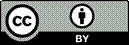Article citation information:

Sága, M., Vaško, M., Handrik, M., Kopas, P. Contribution to random vibration numerical simulation and optimisation of nonlinear mechanical systems. Scientific Journal of Silesian University of Technology. Series Transport. 2019, 103, 143-154. ISSN: 0209-3324. DOI: https://doi.org/10.20858/sjsutst.2019.103.11.

Milan SÁGA, Milan VAŠKO, Marian HANDRIK, Peter KOPAS

CONTRIBUTION TO RANDOM VIBRATION NUMERICAL SIMULATION AND OPTIMISATION OF NONLINEAR MECHANICAL SYSTEMS

Summary. This study considered the solution of the stochastic vibration of non-linear mechanical systems with Gaussian random excitations. It realised a short review of linearisations techniques in stochastic dynamics mainly with application in the area of truss finite element modelling. The presented method of statistical linearisation is applied to numerical testing. In the second part of the article,  the sensitivity analysis of the first two stress statistical moments and structural weight minimising subjected to the random stress constrains presented by mean value and standard deviation was brought to the fore. Cross-sectional areas were used as optimising parameters.

Keywords: statistical linearisation, sensitivity analysis, structural optimising

1. INTRODUCTION

Modelling and analysis of dynamic properties of mechanical systems using linear computational tools are often the first approximation of the actual conduct of the investigated object. The obtained results must be considered with caution, and we must decide whether they are sufficient for us, or we will require more detail and complex study of the issue with the consequent formation of a more appropriate mechanical and mathematical model. If at least one member in the model is nonlinear, then the whole system acts as nonlinear. According to the effect of non-linear members on the dynamic properties, we distinguished systems with significant (strong) and a little significant (weak) nonlinearities. Of course, much more complex are tasks with significant nonlinearities, where the ambiguity of solution causes considerable problems.

The task difficulty may increase if the inputs to a nonlinear system are characterised as a random process or its parameters are random as well, and especially if the investigated processes are non-stationary and non-Gaussian [1,25]. Several different methodical approaches have been developed to solve such problems, applicable only to certain types of tasks. The conditions determining the applicability of individual methods are associated with the type of nonlinearity, its location, as well as the characteristics of random processes operating in the system [1,25].

The most common but simplest nonlinearity is the static transformation (non-inertial), characterised by the following equation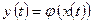.                                                           (1)

Equation (1) presents the functional dependency between the restoring force in a spring and the spring strain. A mechanical system with nonlinearity solved in a static sense is usually a rare phenomenon. In technical practice, we encounter special systems that do not allow neglecting the inertial and dissipative effects. This means that equation (1) in general is no longer a nonlinear algebraic equation, but a nonlinear differential equation. Kropáč in  divides the methods of solving such tasks into the methods of a local nature that well describes the behaviour of a system in a relatively small surrounding of a certain (working) point usually in equilibrium. There are known linearisation approaches that have experienced considerable success in various applications in the engineering practice. In contrast, there are methods of global nature that describe the behaviour of a system in a broader sense, that is, in the entire scope of variables. Solution of a specific task depends on the solvers and their ability to identify the nature of the problem and predict the solution.

As already mentioned, there are a number of independent approaches built on different principles that give acceptable results based on certain assumptions. The global approaches include analysis of nonlinear dynamic systems using the theory of Markov processes, which leads to solving the familiar equation by Fokker-Planck-Kolmogorov (FPK) [2,5]. In practice, we often encounter local approaches represented by the method of statistical linearisation in various modifications (Krylov, Bogoliubov, Caughey, Bolotin , Kazakov , Nigam , Roberts, Spanos [18,23], Elishakoff ), method of statistical quadratisation (Spanos, Donley ), functional method by Volterra and Wiener [14,24]. In addition to the above-mentioned methods, which have been the most discussed ones in the recent decade, other methods have been developed in the past, such as the asymptotic method by Krylov-Bogoliubov-Mitropolsky [2,12], suitably adapted methods of the small parameter, especially the perturbation version , harmonic linearisation  and mean values . Thanks to computer technology also other methods have been given a green light, based on simple but time-consuming and computationally intensive approaches. In particular, there are simulation methods in various modifications. Simulation approaches solve dynamic tasks directly in the time domain, which is demanding on computation time. If we add to the above the random nature of excitation and the need to obtain a complete picture of system behaviour for various excitation realisations, then we come to the Monte Carlo method [1,10,19,20]. Other authors have explored ways of increasing efficiency by combining the Monte Carlo method with the other aforementioned methods (for example, a combination of statistical linearisation and the Monte Carlo method ).

2. METHOD OF EQUIVALENT STATISTICAL LINEARISATION

Equivalent statistical linearisation (ESL) has been used for a relatively long period to deal with randomly excited nonlinear systems, especially in the frequency domain. It is an approximation method, in which solving a system of nonlinear differential equations is replaced by solving an equivalent linear system suitable to obtain the Fourier transform of the system. The method was first presented by Krylov and Bogoliubov [2,12], and further elaborated by Booton [1,2], Caughey , Kazakov , Iwan, Yang , Spanos , then evaluated by Roberts and Spanos in . The development of ESL within nonlinear stochastic dynamics is also associated with the works of Foeter , Malhotra and Penzien , Iwan and Yang , Atalik and Utku , Iwan and Mason , Brücker and Lin , as well as many others. Especially, the 1960s to 1980s were a period of enormous increase in method applications.

We obtain the parameters of an equivalent linear system by satisfying a certain pre-selected criterion, under which we assess the conformity of the original and linearised model especially in the outputs that are characterised mainly by statistical moments. By their very nature, the presented techniques are a generalisation of deterministic linearisation methods by Krylov and Bogoliubov. The most frequently used criteria include:

·      criterion based on energy balance of an actual and equivalent model,

·      criterion of conformity of the corresponding mean values and response dispersions of the original and linearised nonlinear function with a random input (less suitable),

·      criterion of the minimum root-mean-square deviation of an actual and approximated (linearised, or squared) function.

It should be noted that, on one hand, the advantages of this method certainly include its simplicity and admissible estimate of the first two statistical moments, but on the other hand, the change in nature of the random variable in the output, for example, the Gaussian output does not correspond to the Gaussian input, as contemplated by the method’s theoretical foundations. A significant shortcoming of ESL is the possible incompatibility in the spectral response of an actual and linearised system (for example, the difference in the characteristics of the function of power spectral density, etc.).

For a system with multiple degrees of freedom, we present a generalised solution methodology based on the procedure applied by Spanos and Donley in  to the method of equivalent statistical quadratisation. Let’s consider a system of nonlinear differential equations in the form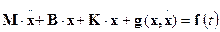,                                           (2)

where M, B, K are the matrices of mass, damping and stiffness of the linear part of the system, x(t) is the vector of unknown displacements, f(t) is the vector of random excitation and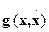is the vector of nonlinear forces that are a function of velocity and displacement. Let’s assume the solution,                                                           (3)

where mx is the vector of displacement mean values, xc is the vector of stationary centered displacements that, however, in general do not necessarily have Gaussian character. If we substitute (3) into (2), we obtain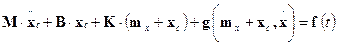.                             (4)

We calculate the vector of the system response mean values as follows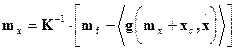,                                         (5)

where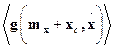is the mean value of the vector of nonlinear forces, and mf is the vector of the mean values of the load forces vector f(t). The load forces vector f(t) can be expressed in the form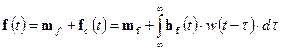,                                    (6)

where fc(t) is the centred load component, of which we assume to have a Gaussian character, w(t) is the above-mentioned centred random function with a “white noise” character. Then, the excitation power spectral density matrix is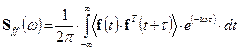or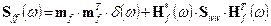,  (7,8)

where (*) is the symbol for a complex conjugate (matrix), Sww is the power spectral density of the function w(t) = 2p×d(t) and is equal to 1, H(w) is the Fourier transform of the impulse function hf (t). The response centred component can be determined from the equation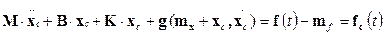.                       (9)

We replace this "original" nonlinear system with an "equivalent linear" system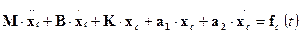,                               (10)

where ai are the equivalent linearisation matrices that we determine from the condition of minimum root-mean-square deviation of differences between the functional values of the actual and linearised function, that is.                                                         (11)

Parameter e is thus the vector of residuals defined as follows.                            (12)

By substitution of (12) to the condition (11) we obtain the system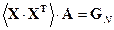,                                                       (13)

where the vectorhas dimensions 2n´1, the symbol < > represents again the mean value operator, A is the matrix of linearisation coefficients a1 and a2 with dimension 2n´n in the form(14)

and the matrix of right-hand sides GN has dimension 2n´n and the form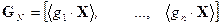.                                         (15)

The system (13) represents n linear systems with 2n unknowns. The resulting equivalent system for centred component will be in the form (10).

In technical practice, we often encounter tasks where input to the system is the power spectral density (PSD) matrix of excitation. Then, we calculate the response according to known equations for linear systems, that is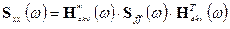,                                        (16)

where the frequency response of a linearised system is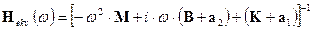,                              (17)

the PSD input matrix is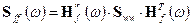(18)

and Sxx(w) will naturally be the PSD output (response) matrix, which determines the spectral properties of the system and by its integration we determine an estimate of second-order statistical moments (variance). The mean value is determined from (5). The actual implementation of the calculation can only be carried out iteratively, since the matrices a1 and a2 contain information about the first two statistical moments. The task leads to solving a system of 2n nonlinear equations, that is to (5) and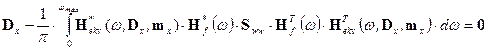,        (19)

where Dx is the matrix of variances (we are mainly interested in diagonal elements). It is usually challenging to perform the initial estimate of mx and Dx, therefore it is preferable to first carry out the calculation only with the linear part of the system, and then calculate the matrices a1 and a2 from the results obtained. These matrices then get more specific in each iterative step. The calculation is completed when the pre-defined convergence criterion is satisfied.

3. STATISTICAL LINEARISATION OF GEOMETRICALLY NONLINEAR ROD FINITE ELEMENTS

Our aim is to present possible ways of analysing geometrically nonlinear rod systems excited by random forces. First, for simplicity, we expressed the total Lagrange’s formulation of geometrical nonlinearity of a plane rod (truss) according to [3,26]. We know that for relative elongation the following relation applies,                                                  (20)

where ue = [u1u2u3u4] is the unknown nodal displacement vector, the matrices BL and BNL have the form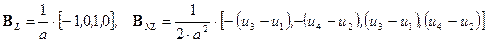,        (21)

where a is the rod (truss) length. Internal forces in the element are expressed as follows.   (22)

Let V be the rod volume (V = const.), s is the normal stress in the element, Ke is the nonlinear rod stiffness matrix, fv = [fv1, fv2, fv3, fv4]T is the vector of internal forces, for which applies that fv3 = -fv1 and fv4 = -fv2. Then, the forces fv1 and fv2 will be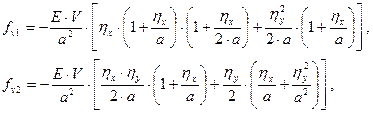(23)

where hx = u3 - u1 and hx = u4 - u2. Nonlinear relations (23) must be “linearised” in accordance with the statistical linearisation method, that is, create a linear equivalent model. We are building on the theoretical basis described in the previous subchapter. Let us resolve the vectors of displacement and internal forces into a unidirectional component and a centred random component, that is.                           (24)

If we apply the criterion (11), we obtain a system of equations (13) that in our case is as follows.          (25)

We solve the system (25) numerically, which generalises the procedure also for application to other types of finite elements with the only difference that the dimension of matrices will change. In simpler cases, such as ours, (Kekv)e can be determined analytically, as presented in  by Cherng and Wen. They expressed the internal forces using the following equivalent relations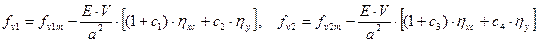(26)

with the ultimate notation of the equivalent relation between the internal forces vector fv and the displacement vector u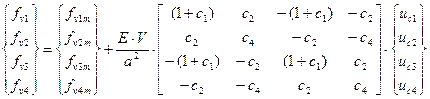(27)

or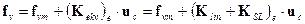.                               (28)

The matrices Klin and KSL represent the linear and linearised component of the resulting stiffness matrix of plane rod element. We calculate the mean values fv1m and fv2m using the following equations (29) and (30)(29)(30)

The linearisation coefficients will be as follows:

c= [6a.hxm + 3E(hx2) + 3E(hy2)] / 2a2,                c2 = [a.hym + E(hxhy)] / a2,

c3 = [a.hym + E(hxhy)] / a2,                        c4 = [2a.hxm + E(hx2) + 3E(hy2)] / 2a2.

After applying globalisation "FEM" procedures to compile linearised equations of motion we obtain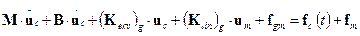.                        (31)

By dividing the equation (6.82) into unidirectional and centred alternating parts we obtain a system of equations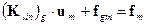and.          (32, 33)

From the theory of stochastic processes for systems with Gaussian distribution [4,8], and provided that the input power spectral density (PSD) Sff (w) is known, we can rewrite the equation of state (33) into the form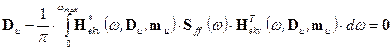,                    (34)

where the equivalent transfer is calculated as follows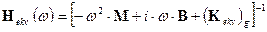(35)

and B is the Rayleigh damping matrix (B = a.M + b.K). The above procedure was based on the total Lagrange formulation of geometric nonlinearity defined for a rod (truss) element. However, it is generally known that the effect of nonlinearity under consideration is much greater in the case of hyper-elastic materials than in the case of metal materials, where the nonlinear component has a character of "weak nonlinearity".

4. SENSITIVITY ANALYSIS AND OPTIMISATION

Optimal design in terms of selected properties is an important part of solving problems in machine mechanics. Most often, it is a standard strength design, which is not always sufficient, even in some cases insufficient. The optimal design may also include spectral tuning [8,11,15], or a design from the perspective of other dynamic system properties [7,14]. A vast development of optimum design of mainly linear problems in continuum mechanics was noted in the 1980s . Optimisation is on the decline in recent years and the main focus is on the optimal design of nonlinear systems.

Therefore, let us consider thinking about optimising the geometrically nonlinear rod (truss) structures with random inputs, sensitivity analysis , and the suitability of some optimisation approaches. The part of many software systems created mainly on the basis of FEM is sensitivity analysis. It is part of the gradient optimisation strategy of all types. Gradient information always tells us more about the impact or significance of each optimisation variable on the monitored mechanical quantities (displacements, stresses, accelerations, etc.). The sensitivity analysis process can be considered as a selection process for the subsequent calculation of significant optimisation variables [11,17]. It is the calculation of gradients by exact way or by apn proximation procedure. In the first case, the gradient or the gradient matrix (sensitivity matrix) is expressed exactly. In the second case, the relevant derivations are calculated using known numerical equations.

Nonlinearity brings considerable complexity to this process, especially in the case of analytical expression of the necessary derivation. Let us show how the problems in the case of stress sensitivity analysis of the truss element are mentioned.

We are searching a derivation of the average stress in the j-th element according to the i-th cross-sectional area (optimisation variable Xi)(36)

where the derivation of fv3mj = - fv1mj according to Xi is generally obtained by derivation of (29). The calculation of the stress dispersion derivation in the j-th element of i-th cross-sectional area will be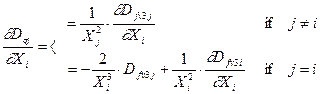(37)

where the derivative of the variance Dfv3j according to Xi is generally obtained by deriving the matrix of dispersions of the internal forces of the j-th element(38)

Since (Kekv)j is a function of the displacement dispersion matrix (Du)j and the average values of the displacement vector (um)j, it is a very complex task. The centre of the presented analysis will be the calculation of the global vector derivation of the displacements average values um and the global matrix of displacements variance Du according to Xi. We obtain a system of nonlinear algebraic equations by derivation of state equations (5 and 34)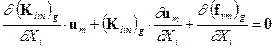,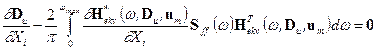.                      (39)

The system of equations (39) can only be solved iteratively, which of course reduces the effectiveness of this approach. The advantage of exact procedures in sensitivity analysis, based on a smaller number of numerical analyses loses the timeliness, hence, numerical derivation is more appropriate.

5. NUMERICAL EXAMPLE

We will design the cross-sectional areas of the rod structure considering three optimisation groups of the same cross-section. The first group of elements consists of rods 8 to 14 with cross-sectional area A1, second rods 15 to 25 with area A2 and third rods 1 to 7 with area A3. The loading force F has a random character of frequency-limited "Gaussian white noise" with PSD: Sff = 15·106 [N2.s] and with average value Fm = 1·105 [N]. The solution will be implemented in the frequency range á0,300ñ [Hz]. Other calculation parameters: material damping coefficient bc = 10-6, Young modulus E = 2,1·1011 [Pa] and dimensions of the construction are a = 0.8 [m], b = 0.8 [m].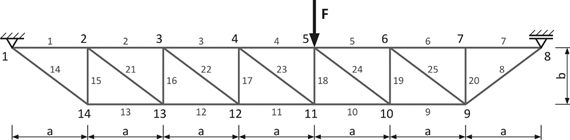Fig. 1. Analysed rod (truss) structure

Solving task was formulated as an optimisation problem with stress restrictions. Three optimisation variables A1, A2, A3 and the objective function (mass of the structure) were considered in the form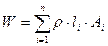,

where n is the number of rods, r is the density of the material, li is the length of the i-th rod, Ai is the cross-sectional area of the i-th rod with a value of A1, A2 or A3, depending on which optimisation group it belongs to.

The restrictive conditions were bound to the first two statistical moments of stresses in the elements with the following values smdov = 50 [MPa] for the average values of stresses in the elements and sDdov = 60 [MPa] for the standard deviations. The mathematical notation of the restrictive conditions is as follows:

for average values:, where,

where sm1, sm2, ..., sm25 are absolute values of the average stresses in the individual elements.

for standard deviations: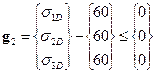, where,

where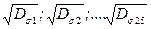are standard deviations of stresses in the individual elements.

The Nelder-Mead optimisation algorithm built in MATLAB was used to solve our optimising problem. Selected results of the optimisation process are processed in Tables 1 to 4 and graphically in Figure 2.

Tab. 1

Objective function

 Construction weight Start value Result value W [kg] 1052.3 453.6

Tab. 2

Design variables for individual optimisation groups

 optimisation variable Start value Result value A1 [m2] 6.0·10-3 3.51·10-3 A2 [m2] 6.0·10-3 1.63·10-3 A3 [m2] 6.0·10-3 3.43·10-3

Tab. 3

Maximum of average stress values for each optimisation group

 Average stress Start value Result value s1m [MPa] 30.21 49.89 s2m [MPa] 14.25 50.13 s3m [MPa] 30.22 50.21

Tab. 4

Maximum of standard deviations of stresses calculated for individual optimisation groups

 Standard deviation of stress Start value Result value s1D [MPa] 39.79 57.62 s2D [MPa] 24.53 54.17 s3D [MPa] 38.47 55.83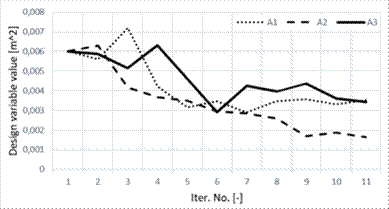Fig. 2. Analysed rod (truss) structure

Minor changes in stress distribution not only for average values but also for standard deviations can be determined from processed results. This was caused by the applied geometric nonlinearity as well as the optimisation process itself as documented in Tables 3 and 4. Consideration of nonlinear analysis computationally complicated the task, but results do not differ significantly from linear stochastic analysis. The reason may be that the geometric nonlinearity of oscillating rod structures does not appear significantly as in other cases.

6. CONCLUSION

Methods of nonlinear analysis and optimisation of mechanical systems are certainly a topical issue today. Many approaches based on simplifying assumptions of different importance have been suggested in the past. Some approaches, especially from the numerical point of view, have succeeded, some have lost importance. This also happened in stochastic nonlinear dynamics. Due to the strong hardware support, time-based Monte Carlo simulations  are now preferred, but the application of linearisation techniques remains a useful tool for frequency domain solutions. The approaches of statistical linearisation are an interesting alternative in connection with finite elements with geometric or material nonlinearity.

Structural optimisation is an essential part of creating and analysing virtual models of structures in construction, engineering or other industries. The results of the presented study confirm that the optimum design of nonlinear model parameters is specific compared to linear models. Nonlinearities can cause significant changes not only in the optimisation process itself , but also in the end results, overestimating or underestimating the first two statistical moments. In conclusion, the optimal design of a nonlinear mechanical system leads to the striking result that cannot be predicted in advance. Therefore, a basic linear analysis of simplified physical models alone cannot be relied upon in design. It is necessary to get as close as possible to reality and it is often non-linear.

Acknowledgement

This work was supported by the grant agency KEGA project No. 015ŽU-4/2017 and grant agency VEGA project No. 1/0073/19.

References

1.             Bendat Julius S. 1990. Nonlinear system analysis and identification from random data. New York: John Wiley & Sons.

2.             Brepta Rudolf, Ladislav Půst, František Turek. 1994. Mechanical vibration. Technical handbook. Praha: Sobotáles.

3.             Cherng Rwey-Hua., Wen Yi-Kwei. 1991. Stochastic finite element analysis of non-linear plane trusses”. Int. Journal Non-Linear Mechanics 26(6): 835-849.

4.             Dekýš Vladimír, Alžbeta Sapietová, Ondrej Števka. 2014. Understanding of the dynamical properties of machines based on the interpretation of spectral measurements and FRF”. Experimental stress analysis 51. Applied Mech. and Materials 486: 106-112.

5.             Dobiáš Ivan. 1988. Nonlinear dynamic systems with random inputs. Praha: Academia.

6.             Dodok Tomáš, Nadežda Čuboňová, Miroslav Císar, Ivan Kuric, Ivan Zajačko. 2017. Utilization of strategies to generate and optimize machining sequences in CAD/CAM”. Procedia Engineering 192: 113-118. ISSN 1877-7058.

7.             Elishakoff Isaac, Pierluigi Colombi. 1993. Successful combination of the stochastic linearization and Monte Carlo methods”. J. of Sound and Vibration 160(3): 554-558.

8.             Frankovský Peter, Darina Hroncová, Ingrid Delyová, Peter Hudák. 2012. Inverse and forward dynamic analysis of two link manipulator”. Procedia Engineering 48: 158-163.

9.             Flizikowski Jozef, Marek Macko, Jacek Czerniak., Adam Mroziński. 2011. Implementation of genetic algorithms into development of mechatronic multi-edge's grinder design”, In ASME 2011 International Mechanical Engineering Congress and Exposition, IMECE. Nov. 11-17, 2011, Denver, Colorado, USA.

10.         Gerlici Juraj, Tomáš Lack. 2014. Modified HHT Method for vehicle vibration analysis in time domain utilisation”. Applied Mechanics and Materials 486: 396-405.

11.         Homišin Jaroslav, Robert Grega, Peter Kaššay, Gabriel Fedorko, Vieroslav Molnár. 2019.Removal of systematic failure of belt conveyor drive by reducing vibrations”. Engineering Failure Analysis 99: 192-202. ISSN 1350-6307.

12.         Kazakov Igor J. 1983. Analysis of the stochastic system in the space of states. Moskow: Nauka.

13.         Krawiec Piotr, Grzegorz Domek, Łukasz Warguła, Konrad Waluś, Jarosław Adamiec. 2018. The application of the optical system ATOS II for rapid prototyping methods of non-classical models of cogbelt pulleys”. MATEC Web of Conferences 157 (01010).

14.         Kropáč Oldřich. 1987. Random effects in mechanical systems. Prague: SNTL.

15.         Mazurkiewicz D. 2010. „Tests of extendability and strength of adhesive-sealed joints in the context of developing a computer system for monitoring the condition of belt joints during conveyor operation”. Eksploatacja i Niezawodnosc – Maintenance and Reliability 3: 34-39.

16.         Nigam N.C. 1983. Introduction to Random Vibrations. Cambridge : MIT Press.

17.         Olsen Gregory R., Vanderplaats Garret N. 1989. Method for Nonlinear Optimization with Discrete Design Variables”. AIAA Journal 27(11): 1584-1589.

18.         Roberts John B., Pol D. Spanos. 1990. Random Vibrations and Statistical Linearization. New York: John Wiley & Sons.

19.         Shinozuka Masanobu. 1972. Monte Carlo simulation of structural dynamics”. Computer and Structures 2: 865-874.

20.         Smutny J., V. Nohal, D. Vukusicova, H. Seelmann. 2018. “Vibration analysis by the Wigner-Ville transformation method”. Komunikacie 4. ISSN: 1335-4205.

21.         Soong T.T., M. Grigoriu. 1993. Random Vibration of Mechanical and Structural Systems. Prentice-Hall: Englewood Cliffs.

22.         Spanos Pol D., M.G. Donley. 1991. Equivalent statistical quadratization for nonlinear systems”. Journal of Engineering Mechanics ASCE 117: 1289-1309.

23.         Spanos Pol D. 1981. Stochastic linearization in structural dynamics”. Applied Mechanics Reviews 34(1): 1-8.

24.         To Cho W.S. 2000. Nonlinear Random Vibration: Analytical Techniques and Applications. Lise, Netherlands: Swets & Zeitlinger B.V.

25.         Turygin Yuri, Pavol Božek, Ivan V. Abramov, Yury R. Nikitin. 2018. Reliability determination and diagnostics of a mechatronic system”. Advances in Science and Technology Research Journal 12(2): 274-290.

26.         Wen Y.K. 1989. Methods of random vibration for inelastic structures”. Applied Mechanics Reviews 42: 39-52.

27.         Zul'ová L., R. Grega, J. Krajňák. 2017. „Optimization of noisiness of mechanical system by using a pneumatic tuner during a failure of piston machine”. Engineering Failure Analysis 79: 845-851. ISSN 1350-6307.

Received 05.01.2019; accepted in revised form 04.05.2019